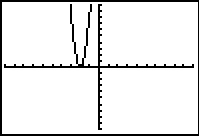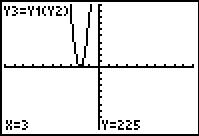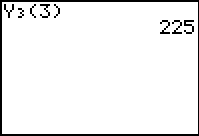Composition of Functions
 The graphing calculator can be used to investigate the composition of functions (composite functions).

 Working with composite functions: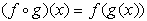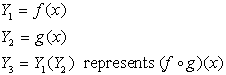* Remember that you can find the Y-subscsripted variables under the VARS key: arrow right to Y-VARS, and choose 1. Function.

Example:

 Given: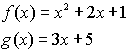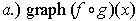Solution: 1.  Enter f (x) into Y1 and g(x) into Y2. 2.  Enter the expression for composition into Y3.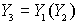3.  Turn off the first two entries so that their graphs      do not appear when the composition is graphed. 4.  Set an appropriate viewing window for the graph. 5.  Graph.  (this will be the answer to part a) 6.  While on the graphing screen, hit TRACE.  Simply      enter the number you wish to find (if that number      is within the viewing window.)  Hit ENTER for the      answer.   (this will be the answer to part b) OR      From the home screen, enter Y3( 3 ), assuming       your functions are entered in Y1 and Y2 with the       composition expression in Y3.       Hit ENTER. (this will be the answer to part b)